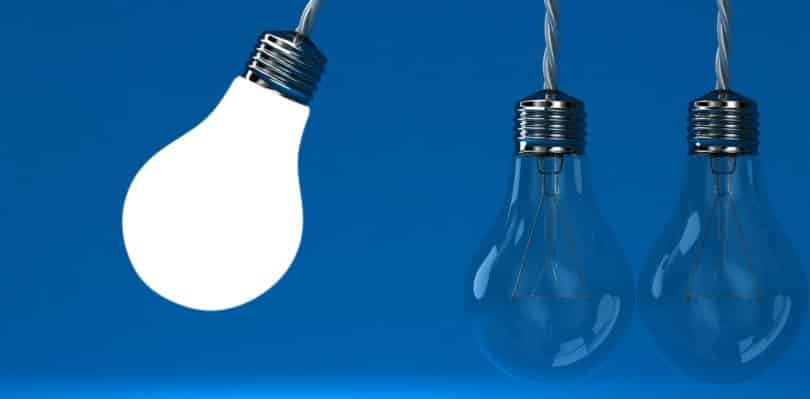CAT sample papers

Basic Arithmetic Operations Online MCQsMathematical Operations Quiz

 Question 1
81038 – ? = 61038
 A 20100 B 20000 C 19099 D 19999
 Question 2
92431 + 64273 + 10428 = ?
 A 167132 B 176134 C 177232 D 168282
 Question 3
Given interchanges : Signs + and × and numbers 4 and 5.

Related: Number series questions

 A 5 × 4 + 20 = 40 B 5 × 4 + 20 = 95 C 5 × 4 + 20 = 104 D 5 × 4 + 20 = 85
 Question 4
4628 – 954 – 1253 = ?
 A 1029 B 2421 C 4232 D 3513
 Question 5
8888 + 888 + 88 + 8 = ?
 A 9872 B 9962 C 10072 D 8962
 Question 6
282828280 / 14 = ?
 A 20202 B 2020220 C 20202020 D 202020
 Question 7
0.999999 / 0.011 = ?
 A 90.908 B 9.0908 C 909.08 D 90.909
 Question 8
43488 – 34567 + 14368 = ?
 A 13289 B 22289 C 24289 D 23289
 Question 9
If + means ÷, – means ×, ÷ means +, and × means –, then 48 + 12 ÷ 15 × 2 – 5 = ?
 A 9 B 3 C 18 D 8
 Question 10
Which one of the four interchanges in signs and numbers would make the given equation correct? 6 × 4 + 2 = 16
 A None B + and ×, 2 and 6 C + and ×, 4 and 6 D + and ×, 2 and 4
 Question 11
÷ means +, × means –, + means × and – means ÷ then 15 – 5 + 2 × 3 ÷ 2 = ?
 A 36 B 5 C 4 D 13
 Question 12
13025 + 1019 – ? = 7622
 A 7422 B 6422 C 6412 D 7412
 Question 13
Given interchanges : Signs – and × and numbers 3 and 6.
 A 3 × 6 – 4 = 33 B 3 – 6 × 8 = 10 C 6 – 3 × 2 = 9 D 6 × 3 – 4 = 15
 Question 14
879 x 37 x 8 = ?
 A 260184 B 257224 C 32523 D 292707
 Question 15
0.9329 – 0.7321 + 4.329 + 0.002 = ?
 A 4.2514 B 45.318 C 4.5318 D 0.45318
 Question 16
608 x 8 – 48 = ?
 A 24240 B 4800 C 2424 D 4816
 Question 17
Which one of the four interchanges in signs and numbers would make the given equation correct? 3 + 5 – 2 = 4
 A None of these B + and –, 2 and 3 C + and –, 3 and 5 D + and –, 2 and 5
 Question 18
M denotes ×, D denotes ÷, A denotes + , S denotes - Then 25S72D12A1M6 is
 A 2 B 35 C 25 D 20
 Question 19
13284 / 81 = ?

Related: Blood Relations (Reasoning) MCQs

 A 184 B 124 C 165 D 164
 Question 20
\$ means -, * means +, @ means ÷, # means ×, then 3 # 5 * 63 @ 7 \$ 12 = ?
 A 1 B 12 C 52 D 22
 Question 21
15.04 – 0.065 = ?
 A 14.875 B 14.975 C 15.795 D 14.957
 Question 22
6142 + ? = 5139 + 8136
 A 7313 B 6863 C 7033 D 7133
There are 22 questions to complete.